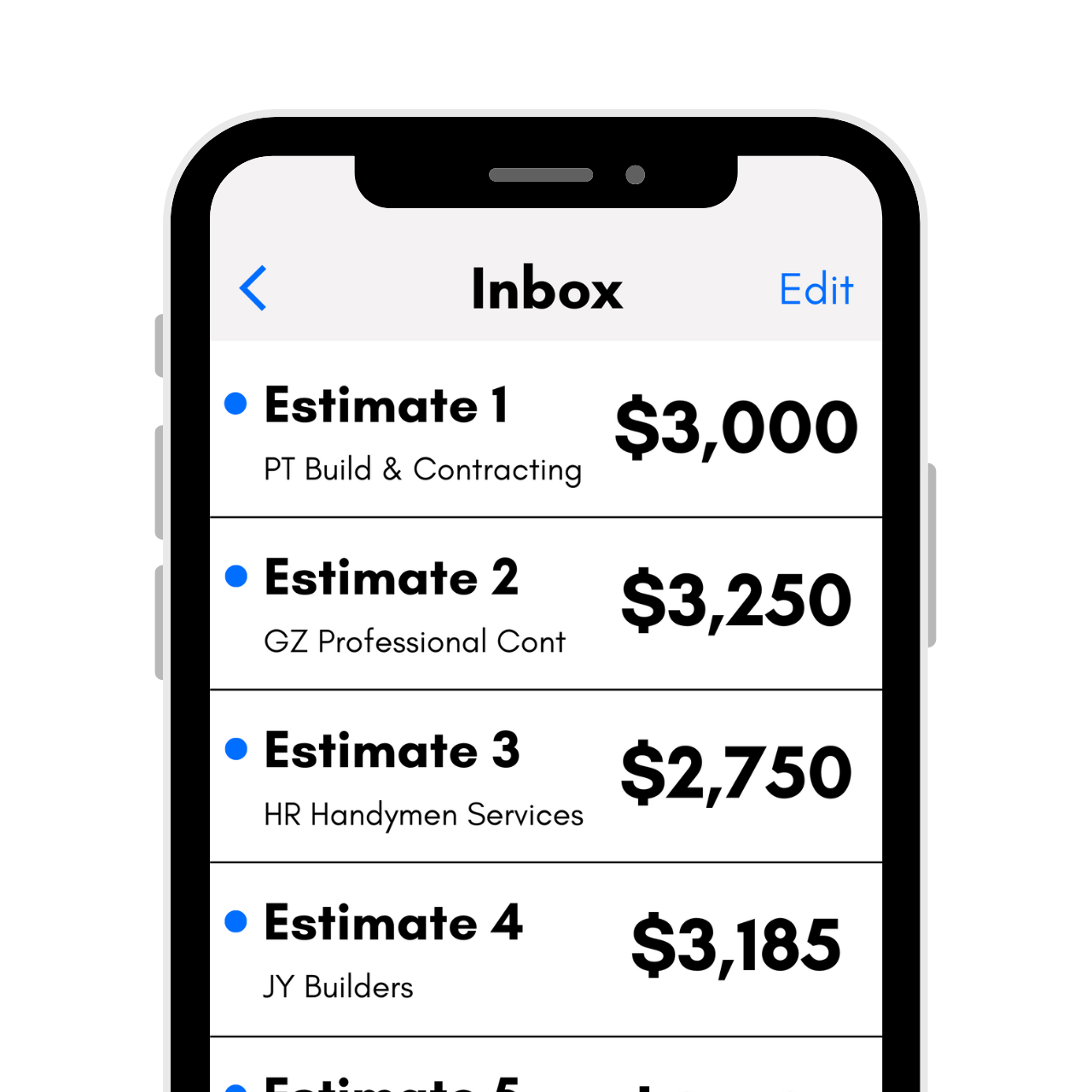# Wallpaper Calculator – Estimate Rolls and Cost

Calculate how many rolls of wallpaper you need for your project by entering your room’s dimensions and coverage per roll below. Estimate the cost by adding the price per roll.

Room Dimensions
usable ft2 per roll
Optionally enter the price per roll
\$

## Wallpaper Estimate:

Wallpaper Needed

rolls
Estimated Material Cost
\$
wallpaper cost
Total Square Footage

sq ft
Learn how we calculated this below

## How to Estimate How Much Wallpaper You NeedWhether you choose to use wallpaper on an accent wall or to cover all the walls of your home, it’s important to understand how much material to order. Dye lots often mean that if you run out and order more later, you may not get an exact match of color between rolls, so ordering the correct amount from the beginning can help ensure your project goes smoothly.

To calculate how much wallpaper you need, follow a few simple steps. First, find the square footage of the surfaces to be wallpapered, determine the usable wallpaper per roll, then calculate how many rolls of wallpaper will be needed to cover the room’s square footage.

Get free estimates from wallpaper pros in your area to compare prices### Step One: Find the Square Footage of the Room

The first step in estimating wallpaper is to find the square footage of the area to be papered. Square footage is the measurement of the area in feet. You can find the square footage by measuring the length and height, and then multiplying them together.

Make sure length, width, and height measurements are in feet before you multiply to find the square footage. It may be easiest to round your measurements to avoid working with inch fractions and decimals.

However, if you are measuring for a wallpaper border, or if you want to estimate the wallpaper for a short section of wall, you can measure in inches; once you get the height and width and multiply for square inches, divide by 144 to get the square feet. Round up to the nearest whole foot.

For example, let’s find the square footage of a wall that is 12 ft wide by 9 ft high.

sq ft = width × height
sq ft = 12 ft × 9 ft
sq ft = 108 sq ft

If your walls are not simple rectangles or are complex, then you can split up the wall into smaller chunks and find the square footage of each part separately, then add the square footage measurements together.

For example, if your wall is not a simple rectangle, break it into different sections and measure the square footage of each section and add the different sections together.

Once you know the square footage of each wall, add all of them together to find the total project square footage.

Here’s a tip: try using our square footage calculator to easily find the square footage of your room.

If you have a lot of windows or doors in the room, you may want to subtract them from your total square footage. Measure the width and height of each window or door in feet, and multiply them together to get its square footage. Subtract the square footage of each area not being papered from the total.

### Step Two: Find the Wallpaper Coverage

The next step is to find the usable coverage for your chosen wallpaper in square feet. Different wallpaper patterns have different amounts of usable square footage, which is determined by the drop match pattern.

Drop match patterns align both horizontally and vertically. They can be full, 1/2 drop or 1/4 drop, depending on the size of the pattern. In order to line up, you need to start the next row of wallpaper a certain distance from the last, by measuring a “drop” on the roll of the recommended number of inches.

When you drop the next section of wallpaper to align the pattern with the previous section, there will be waste that needs to be cut to make the pattern line up. This waste reduces the usable square footage of the roll.

The following are estimates of the coverage of different drop patterns.

Usable wallpaper per roll for various drop patterns. A standard US wallpaper roll with no pattern will cover 36 square feet, and a standard European roll will cover 29 square feet
Drop Pattern Wallpaper Coverage
no drop pattern 29 – 36 sq ft
0″ – 6″ drop pattern 25 sq ft
7″ – 12″ inch drop pattern 22 sq ft
13″ – 18″ inch drop pattern 20 sq ft
19″ – 23″ inch drop pattern 15 sq ft

### Step Three: Calculate Wallpaper Rolls Needed

The final step in estimating wallpaper is to use the total square feet and coverage per roll to find the number of rolls you need. Find the number of rolls needed by dividing the total square footage by the usable square footage per roll.

For example, find the number of rolls needed to cover a room with 350 sq ft of walls using rolls that have 25 usable square feet.

rolls = wall sq. ft. ÷ usable sq. ft.
rolls = 350 sq. ft. ÷ 25 sq. ft.
rolls = 14

We recommend ordering about 10% to 20% extra to allow for cuts and waste material. If you are fairly close to an even roll, then it may be a good idea to order an extra roll to ensure you are not short on material.

It might even be worth ordering some extra to allow for future patches and repairs, if needed.

If you’re also doing some painting in this space, you might be interested in estimating how many gallons of paint you’ll need.